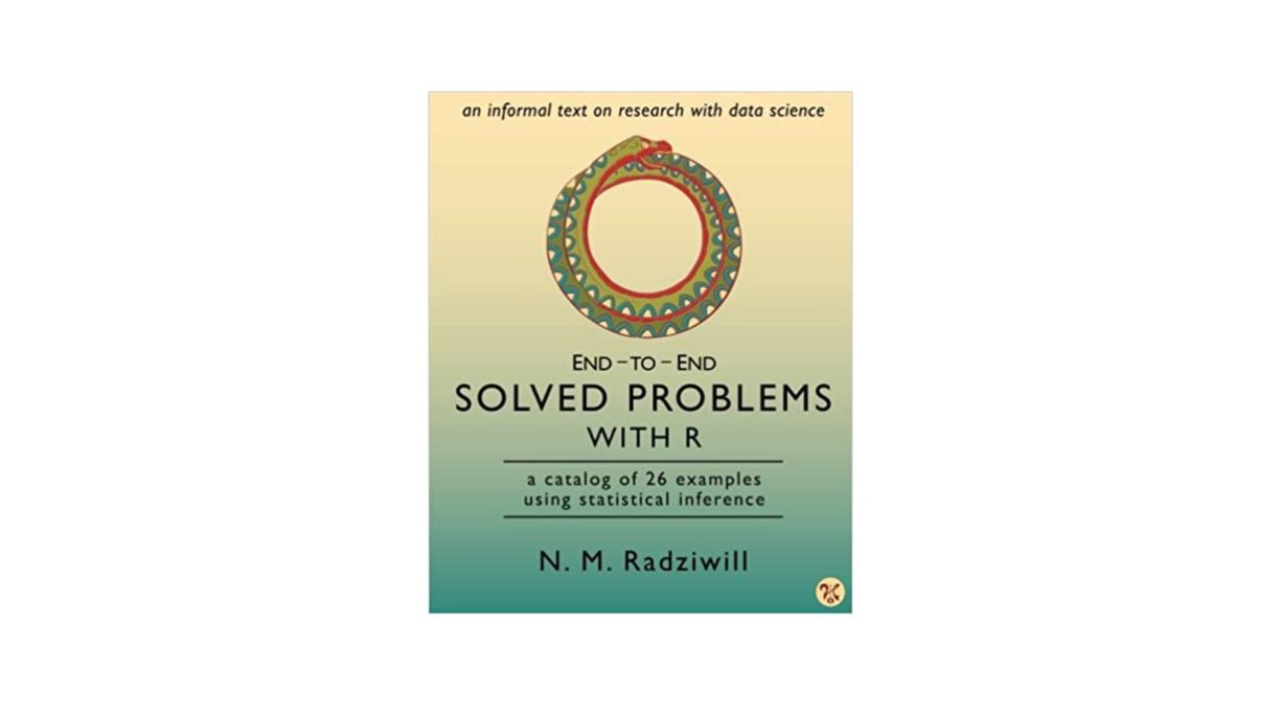# \$12.00 USD or more# End to End Solved Problems With R

### Need a quick, easy, comprehensive intro to research with R? This book is great for high school, undergraduate, and graduate students who need to create and investigate research questions -- for science fairs, projects, a thesis, or a dissertation -- particularly if they're not strong in math.Designed as a companion to Statistics (The Easier Way) With 2, 2nd Ed. (but completely useful standalone) this book contains 26 complete examples of research questions that are solved using basic data science and methods from statistical inference. Each problem is solved analytically (using equations) and computationally (with the R Statistical Software), which helps you understand what’s happening beneath the surface of the statistical software. Estimation and confidence intervals help you check your progress for 13 different inference tests: one sample t-test, two sample t-test (equal variances), two sample t-test (unequal variances), paired t-test, one way analysis of variance (ANOVA), one proportion z-test, two proportion z-test, Chi-square test of independence, Chi-square goodness of fit test, simple linear regression (tests for slope and intercept), multiple regression (test for slopes and intercept), Chi-square test for one variance, and F test for homogeneity of variances.

What you'll get:

• A link to your PDF eBook will be sent to the email address you specify at check out.

Looking for a print copy? Find one on Amazon here.